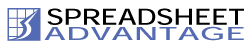Resource Links | Link to us | Sitemap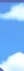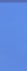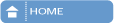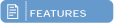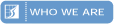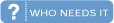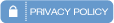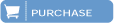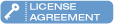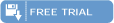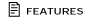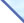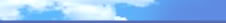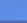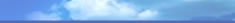Spreadsheet Analysis The Spreadsheet Analysis tool provides useful information on a spreadsheet, helping you to answer questions such as: • Which are the most important or largest worksheets? • How are the worksheets related to each other? How does the logic flow within the spreadsheet? • Are there many blank rows being stored, increasing file size unnecessarily? It produces the following statistics for each worksheet: • The number of unique formulae in the worksheet (a formula copied from the cell above or to the left is not counted again). • The number of unique formulae in the worksheet referring to every other worksheet. • The number of rows and columns in use and the number actually stored in the spreadsheet.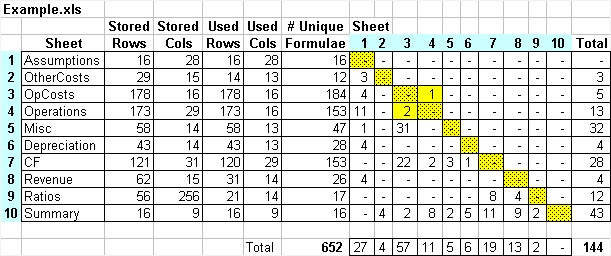For example, the figure above shows that the OtherCosts sheet has 12 unique formulae, 3 of which refer to the Assumptions sheet. The sheet order is arranged so that, as far as possible, each sheet appears after all of the sheets it refers to. This helps you understand the structure and the logic flow of the spreadsheet. (When it is not possible to order certain sheets so that each sheet is after the sheets it refers to, it groups these sheets into a yellow “block”, as was done for the OpCosts and Operations sheets in the figure above.) E-mail: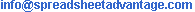Home | Features | Compare Spreadsheets | Spreadsheet Analysis | Who we are | Who needs it | Purchase | Free Trial Copyright © 2005 Spreadsheet Advantage Pty Ltd. All rights reserved. Search Engine Optimisation by One Eleven Media